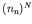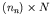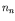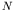Computational & Technology Resources an online resource for computational,engineering & technology publications not logged in - login Computational Science, Engineering & Technology SeriesISSN 1759-3158 CSETS: 15INNOVATION IN ENGINEERING COMPUTATIONAL TECHNOLOGY Edited by: B.H.V. Topping, G. Montero, R. Montenegro Chapter 10Numerical Modelling of Complex Fluids: State-of-the-Art, Recent Developments and New Challenges F. Chinesta*, A. Ammar+, B. Mokdad+ and R. Keunings#*Laboratoire de Mécanique des Systèmes et des Procédés, UMR 8106 CNRS-ENSAM-ESEM, Paris, France +Laboratoire de Rhéologie, INPG, UJF, CNRS (UMR 5520), Grenoble, France #CESAME, Université Catholique de Louvain, Louvain-la-Neuve, Belgium doi:10.4203/csets.15.10 Full Bibliographic Reference for this chapter F. Chinesta, A. Ammar, B. Mokdad, R. Keunings, "Numerical Modelling of Complex Fluids: State-of-the-Art, Recent Developments and New Challenges", in B.H.V. Topping, G. Montero, R. Montenegro, (Editors), "Innovation in Engineering Computational Technology", Saxe-Coburg Publications, Stirlingshire, UK, Chapter 10, pp 193-215, 2006. doi:10.4203/csets.15.10 Keywords: numerical modelling, kinetic theory, Fokker-Planck equation, stochastic simulation, model reduction, separated representation, tensor product spaces. Summary Kinetic theory models involving the Fokker-Planck equation, can be accurately discretised using a mesh support (Finite Elements, Finite Differences, Finite Volumes, Spectral Techniques). However these techniques involve a high number of approximation functions. When the model involves high dimensional spaces (including physical and conformation spaces and time) standard discretisation techniques fail due to the excessive computation time required to perform accurate numerical simulations. Stochastic simulations have been widely used to bypass the difficulty just referred to. However accurate representations of molecular conformation distributions require simulation of an extremely large number of trajectories of the stochastic processes, as well as working with a very small time step. Some new appealing strategies that allow these limitations to be circumvented are based on the use of a reduced approximation basis within an adaptive procedure, some of them making use of an efficient separated representation of the solution. This paper explore the potential of these new advanced strategies. The most frequently used kinetic theory models defined from Fokker-Planck equations have two important particularities: (i) they can be expressed in a separated form (this is the case for multi-bead models) and (ii) in general, realistic molecular models involve springs with finite extension which implies that the distribution function vanishes on the boundary of the domain where the conformation of the spring is defined. In this case, the separated representation and the definition of tensor product approximation spaces, run perfectly, and allow circumvention of the difficulties related to the multidimensional character of kinetic theory models, as proved in . This technique consists of a separated representation of the molecular conformation distribution, that introduced in the variational formulation of the Fokker-Planck equation leads to an iteration procedure that involves at each step the resolution of a small-size non-linear problem. Thus, the number of degrees of freedom involves in the discretisation of the Fokker-Plack equation can be reduced from(required in the usual grid/mesh based strategies) to(being the number of nodes involved in the discretisation of each conformation coordinate andthe dimension of the conformation space). In  we considered the steady state solution of some classes of multidimensional partial differential equations, and in particular those governing the molecular configuration distribution in kinetic theory models of complex fluids. In the conclusion of that paper we claimed that the resolution of multidimensional transient Fokker-Planck equations could be considered within an incremental time discretisation. However, as time is only the same as the other coordinates, one could expect a coupled space-time resolution. The main difficulties related to such an approach lie in the fact that the initial condition is non-zero and the procedure proposed in  cannot be applied in a direct manner. Other difficulties lie in the fact that space approximations are built using standard piece-wise functions, and when this kind of approximation is used to construct time interpolation, a known inconsistency related to centred differences of time derivatives is encountered. In  we propose alternatives to circumvent both difficulties, and then to allow an efficient treatment of multidimensional transient kinetic theory models. The technique proposed in our former work  and extended in  to transient simulations, is, in our opinion, a suitable choice when we are dealing with highly multidimensional parabolic PDE with homogeneous boundary conditions.Despite implications of the apparently negative assumption about its generality, usual molecular descriptions make use of a configuration distribution function defined in a bounded domain and which vanishes on its boundary. References 1 A. Ammar, B. Mokdad, F. Chinesta, R. Keunings, "A fast solver for some classes of multidimensional partial differential equations encountered in kinetic theory modelling of complex fluids". Journal of Non-Newtonian Fluid Mechanics, Submitted. 2 A. Ammar, B. Mokdad, F. Chinesta, "A fast solver for some classes of multidimensional partial differential equations encountered in kinetic theory modelling of complex fluids. Part 2: Transient simulation using space-time separated representations". Journal of Non-Newtonian Fluid Mechanics, Submitted. purchase the full-text of this chapter (price £20) Back to top ©Civil-Comp Limited 2020 - terms & conditions